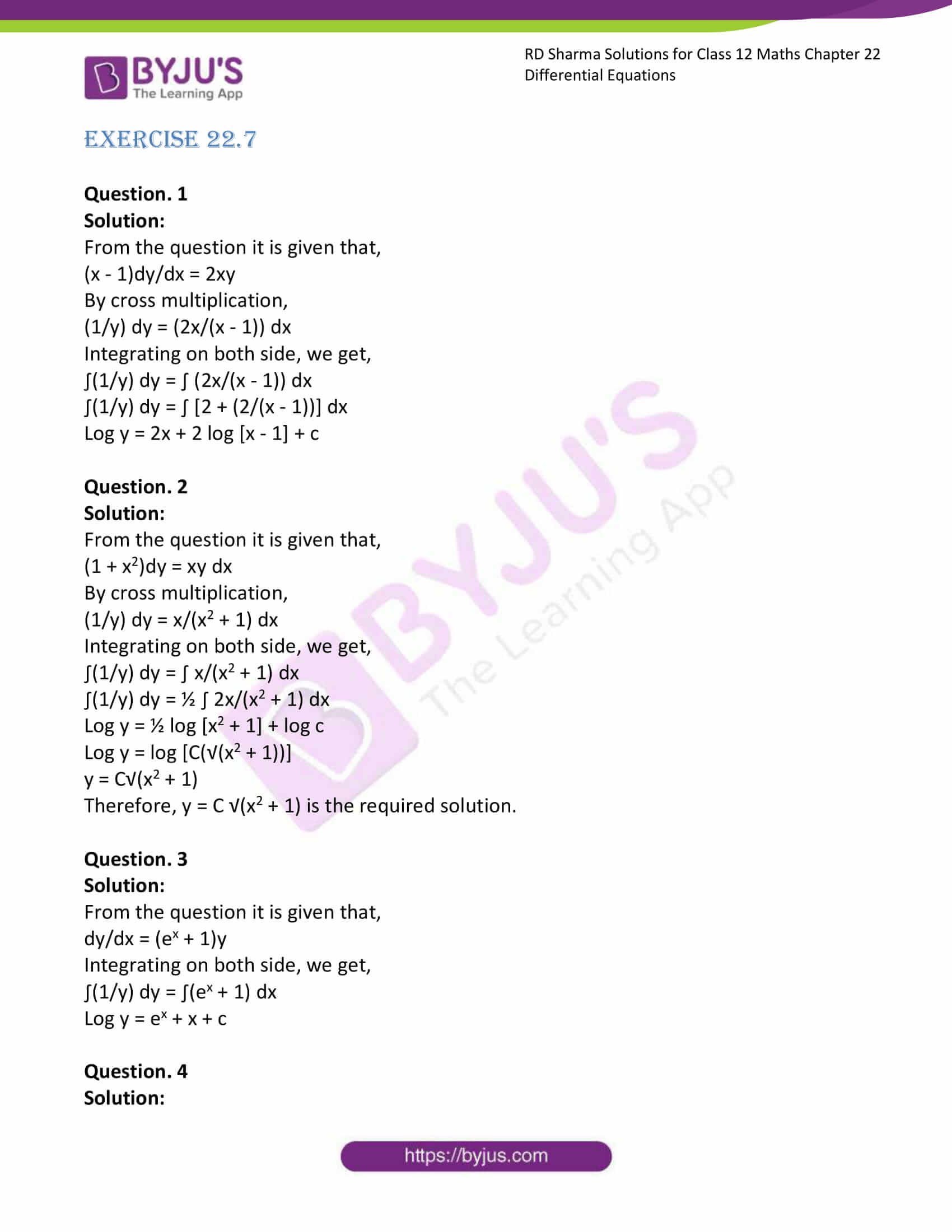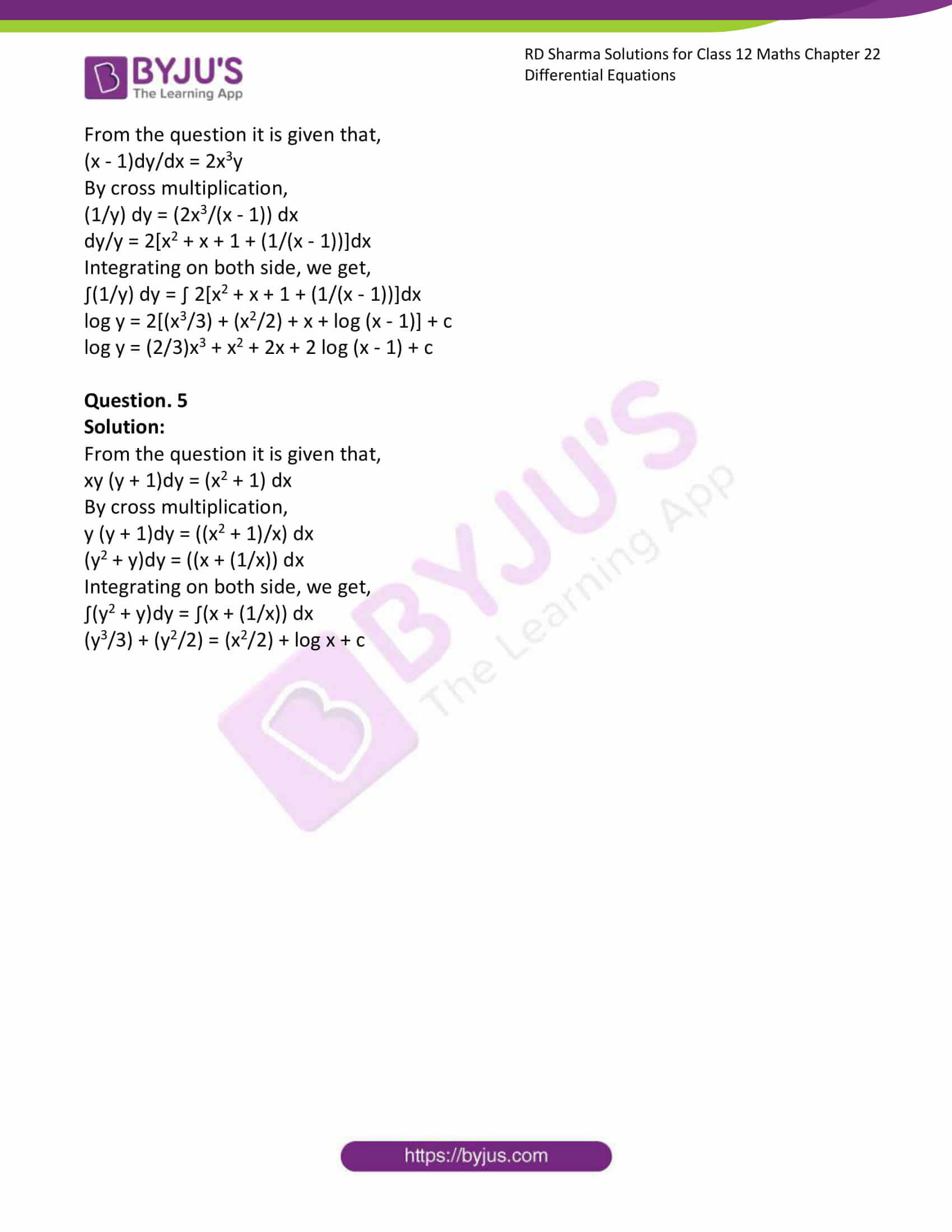# RD Sharma Solutions Class 12 Differential Equations Exercise 22.7

RD Sharma Solutions for Class 12 Maths Exercise 22.7 Chapter 22 Differential Equations are available on this page to provide solutions to the questions present in the exercises. Maths is a subject which requires understanding and reasoning skills accompanied by logic. RD Sharma Solutions for Class 12 Maths Chapter 22 are available in PDF format so that students can download and learn offline as well.

## Download PDF of RD Sharma Solutions for Class 12 Maths Chapter 22 Exercise 7### Access RD Sharma Solutions for Class 12 Maths Chapter 22 Exercise 7

EXERCISE 22.7

Question. 1

Solution:

From the question it is given that,

(x – 1)dy/dx = 2xy

By cross multiplication,

(1/y) dy = (2x/(x – 1)) dx

Integrating on both side, we get,

∫(1/y) dy = ∫ (2x/(x – 1)) dx

∫(1/y) dy = ∫ [2 + (2/(x – 1))] dx

Log y = 2x + 2 log [x – 1] + c

Question. 2

Solution:

From the question it is given that,

(1 + x2)dy = xy dx

By cross multiplication,

(1/y) dy = x/(x2 + 1) dx

Integrating on both side, we get,

∫(1/y) dy = ∫ x/(x2 + 1) dx

∫(1/y) dy = ½ ∫ 2x/(x2 + 1) dx

Log y = ½ log [x2 + 1] + log c

Log y = log [C(√(x2 + 1))]

y = C√(x2 + 1)

Therefore, y = C √(x2 + 1) is the required solution.

Question. 3

Solution:

From the question it is given that,

dy/dx = (ex + 1)y

Integrating on both side, we get,

∫(1/y) dy = ∫(ex + 1) dx

Log y = ex + x + c

Question. 4

Solution:

From the question it is given that,

(x – 1)dy/dx = 2x3y

By cross multiplication,

(1/y) dy = (2x3/(x – 1)) dx

dy/y = 2[x2 + x + 1 + (1/(x – 1))]dx

Integrating on both side, we get,

∫(1/y) dy = ∫ 2[x2 + x + 1 + (1/(x – 1))]dx

log y = 2[(x3/3) + (x2/2) + x + log (x – 1)] + c

log y = (2/3)x3 + x2 + 2x + 2 log (x – 1) + c

Question. 5

Solution:

From the question it is given that,

xy (y + 1)dy = (x2 + 1) dx

By cross multiplication,

y (y + 1)dy = ((x2 + 1)/x) dx

(y2 + y)dy = ((x + (1/x)) dx

Integrating on both side, we get,

∫(y2 + y)dy = ∫(x + (1/x)) dx

(y3/3) + (y2/2) = (x2/2) + log x + c